# Skip Counting by 10

Skip Counting By 10• Skip counting in tens means to keep adding ten to a number.
• A one hundred number grid is a useful way to teach skip counting.
• Every time we add 10, we move one square down the number grid.
• We can count up in tens from 7, adding ten each time.
• We have 7, 17, 27, 37, 47, 57, 67, 77, 87 and 97.
• When skip counting in tens, the numbers will all end in the same digit.
• The digit in the tens column will increase by one each time.

To skip count by 10, add ten to get to the next number.

The digit in the tens column will increase by 1 each time.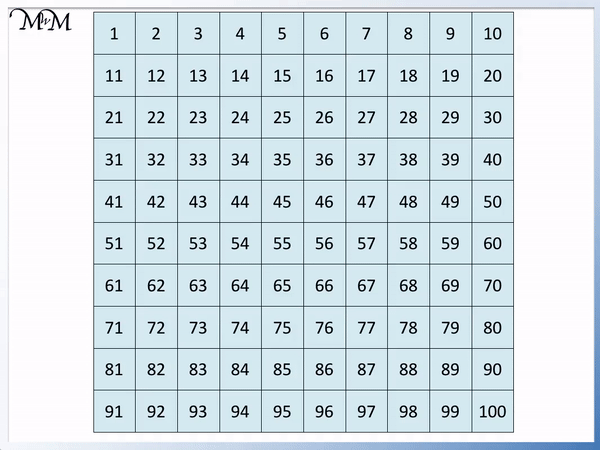• To skip count backwards by 10, subtract 10 each time.
• Subtracting ten on the number grid results in moving one square up each time.
• We will skip count backwards in tens from 42.
• Moving up the number grid, we have 42, 32, 22, 12 and 2.
• Two is less than ten and so we stop here.Supporting Lessons

#### Skip Counting by 10 from Any Number: Accompanying Lesson Worksheet# Skip Counting by 10

## What is Skip Counting by 10?

Skip counting by 10 means to count up in tens, adding ten each time. Do not say the numbers in between. Skip counting in tens is important because it allows us to count up more quickly than counting up in ones.

For example, skip counting in tens from zero we have 10, 20, 30, 40, 50, 60, 70, 80, 90 and 100.

Below is an example of a blank skip counting caterpillar worksheet that you can use to practise skip counting. We will count up in tens to complete the caterpillar, writing a number in each segment.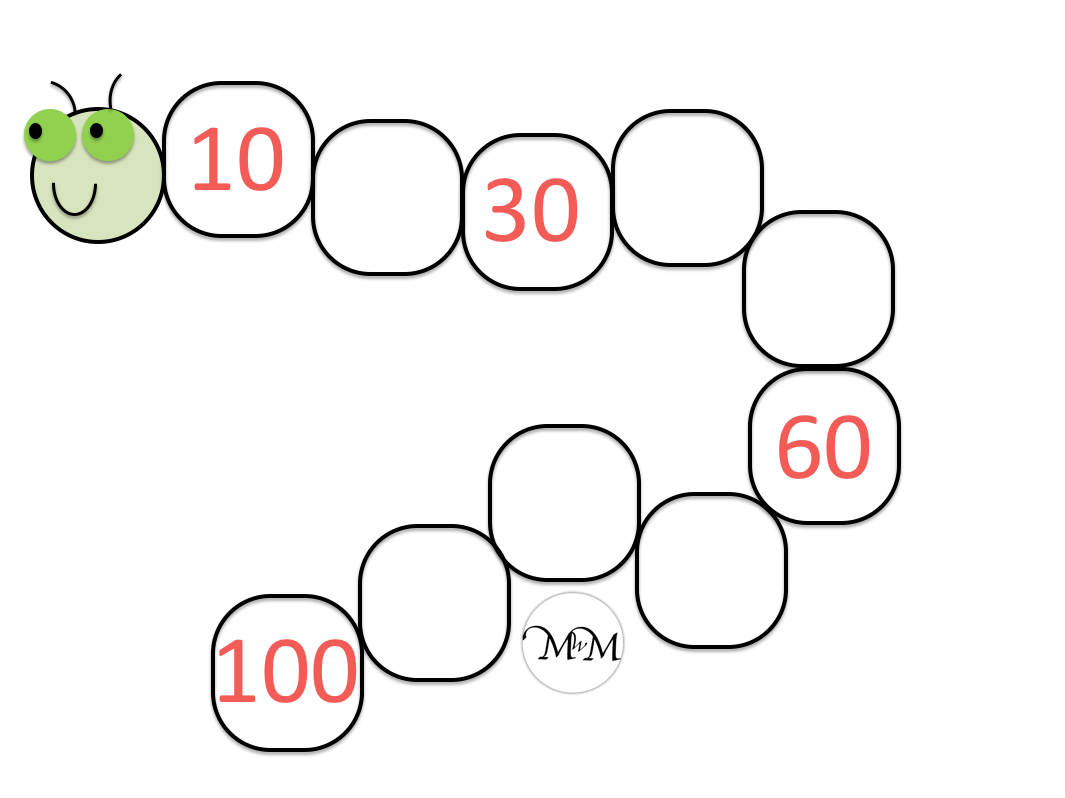Here is the completed caterpillar example.## How to Skip Count by 10

To skip count by 10, add 10 to get to the next number. This is most easily done by increasing the digit in the tens column of the number by 1. For example, skip counting in tens from 7, we have 7, 17, 27, 37, 47, 57, 67, 77, 87, 97.

The tens digit simply increases from 1 to 2 to 3 etc.

An alternative method for skip counting by 10 is to use a number grid to 100 and move down one row each time as you add 10.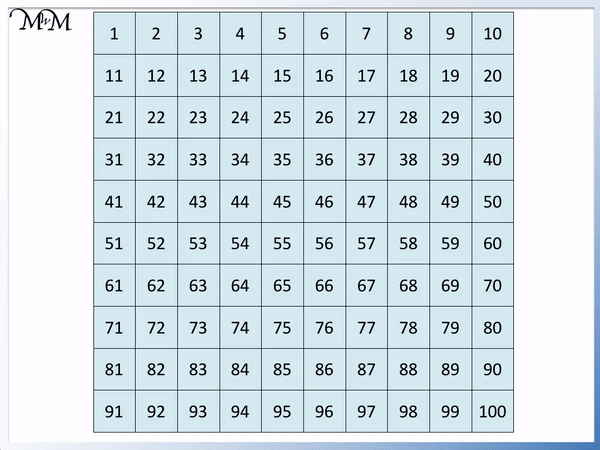We have: 7, 17, 27, 37, 47, 57, 67, 77, 87 and 97.

We stop at 97 because it is the last number in the pattern before 100 but we could keep counting on if we needed to.We can see that the numbers all end in the same digit as the number we started on.

The numbers all end in 7 because we skip counted in tens starting at 7.We simply add 1 more to the tens digit with each addition of 10.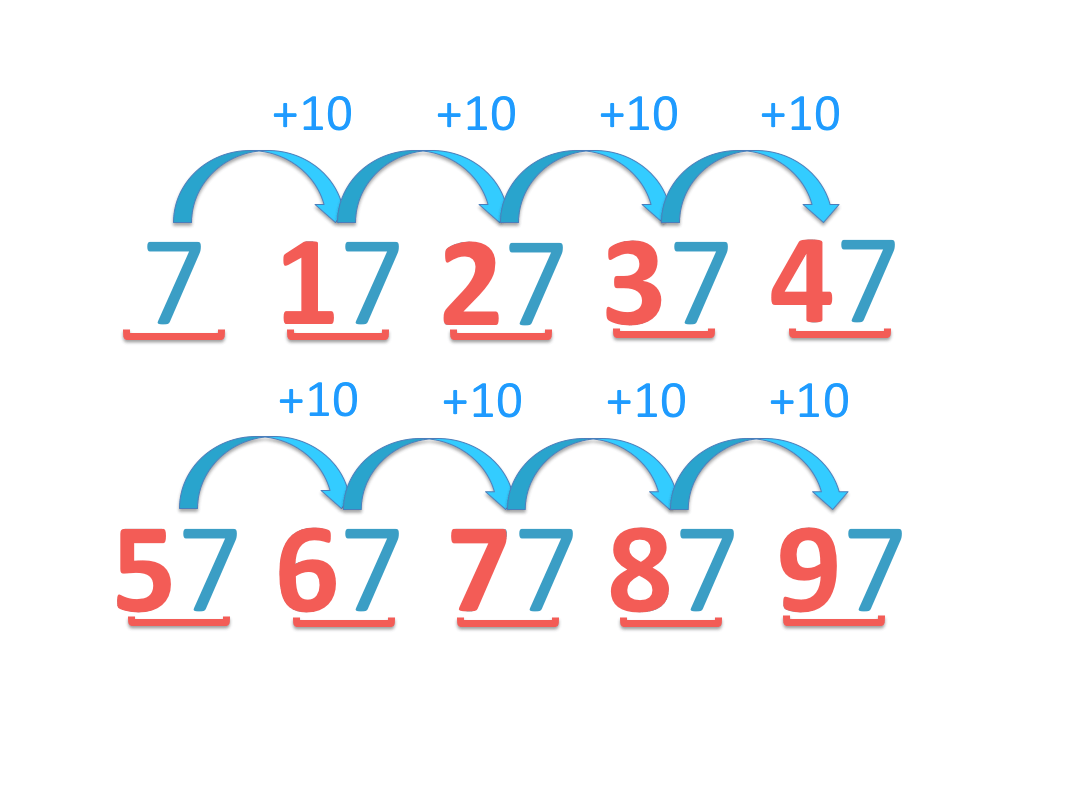## Counting on in Tens from Any Number

To count on in tens from any number, add 10 to get to the next number. The easiest way to do this is to increase the digit in the tens column by 1 each time. For example, starting at 1, we have 11, 21, 31, 41, 51, 61, 71, 81 and 91.

To skip count in tens from any number, simply add one to the tens digit each time. The numbers will always end in the same digit in their ones column.

Remember that to add 10 on a number grid, simply move down one square.

Instead of starting at zero, we start at ‘1’.Moving one square down the grid each time is adding ten. We have:

1, 11, 21, 31, 41, 51, 61, 71, 81 and 91.

We could continue skip counting in tens further if we needed to.We can start at any number on the number grid and move down the column to help us skip count in tens. Remember that this number grid can be a useful teaching tool to help practise counting in tens.

We can choose any number on the number grid to count on in tens from.

For example, here is 46. We will count on in tens from 46.

We will start at 46 and move down from square to square, adding ten each time.Again we simply increase the tens digit by one each time from 46 to 56, to 66 and so on.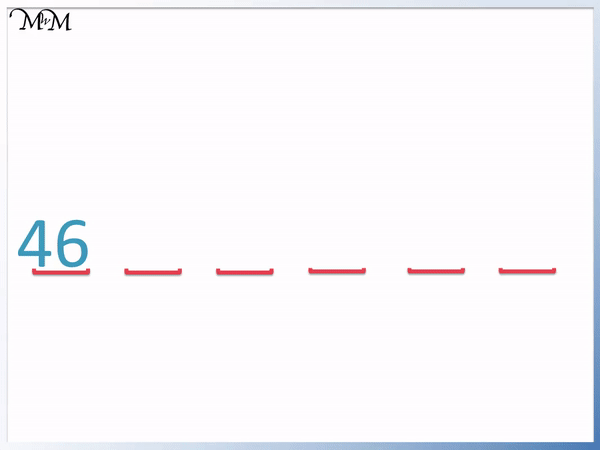46 ends in the digit ‘6’ in its units column and so, the other numbers in our skip counting pattern end in ‘6’ too.

Here is a blank skip counting caterpillar that we will complete together, skip counting up in tens from 11.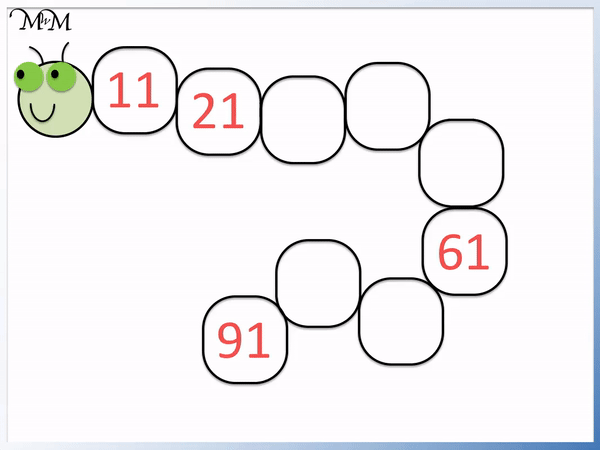11 ends in the units digit of ‘1’ and so, the other numbers in the pattern will also end in a ‘1’.

In the following example, we are counting up in tens starting with 3.

All of the next numbers in the pattern will also end in 3.## Teaching Skip Counting by 10

To teach counting on in tens, it is helpful to use a number grid. Adding ten on a number grid simply involves moving down one row from the original number. Using the number grid it is possible to notice patterns more easily, showing how the digit in the tens column increases by 1 each time.

To skip count in tens, keep adding ten to get to the next number. To easily skip count in ten, increase the tens digit by one each time. The numbers will all end in the same digit in the units column.

Skip counting is a quicker way to count than counting in ones.

When teaching skip counting by 10 for the first time, it can be useful to use a number grid to 100 to help visualise the patterns in the numbers.

Starting from zero, we can add ten to get to 10.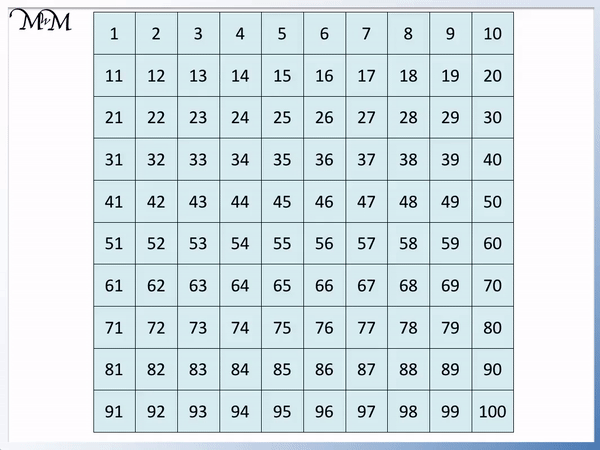We continue to add 10 each time and get: 20, 30, 40, 50, 60, 70, 80, 90 and 100.

We can see that every time that we add ten to a number on the number grid, we move one square downwards.

We started with ‘0’ and counted up in tens. When skip counting by 10 from zero, the numbers are all in the ten times table.

We can see a pattern that all of the numbers end in the same digit in the units column. The numbers all end in zero.When teaching skip counting by 10, it is important to point out that adding ten to a number increases its tens digit by 1 each time.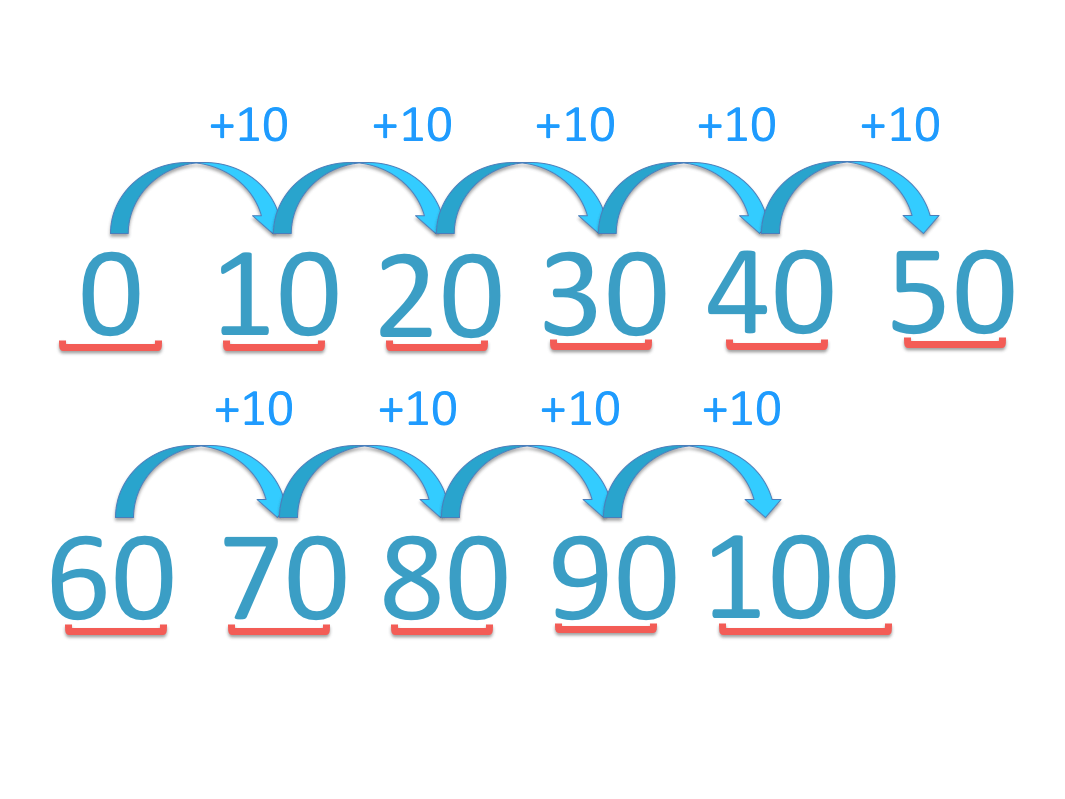We can see the pattern in the tens digits increasing from 1 to 2, to 3 and so on. 100 is 10 tens.

## Skip Counting by 10 Backwards

To skip count backwards by 10, subtract 10 each time. Subtracting 10 on the number grid results in moving one square up each time. The digit in the tens column will decrease by 1 each time.

For example, from 42 counting backwards, we have 42, 32, 22, 12 and 2. The digit in the tens column decreases by 1 each time from 4 to 3 to 2 to 1 to 0.

We can find 42 on the number grid to 100.

To subtract 10 on the number grid, we move up the grid to the square directly above the number we are currently in.42 ends in a ‘2’ and so the other numbers in the pattern will also end in a ‘2’.

Counting backwards, we have 42, 32, 22, 12 and 2.

We do not continue to subtract 10 because 2 is less than 10 and there are no further squares shown above 2 on the number grid.Here is another example.

Skip counting backwards in tens, starting at 100 we have 100, 90, 80, 70, 60, 50, 40, 30, 20, 10, 0.

To skip count backwards in tens subtract ten to get to the next new number. The units column will remain the same but the tens column will decrease by one each time. The numbers will all end in the same digit.We can use skip counting caterpillar worksheets to practise skip counting backwards.

Here is a blank example for skip counting backwards.Here is the caterpillar worksheet being completed by skip counting backwards in tens.We can see that the numbers in the caterpillar worksheet for skip counting backwards are the same as skip counting forwards. Skip counting backwards can be useful for practising the patterns seen when skip counting forwards.When teaching skip counting, it is important to first practise skip counting forwards before moving on to skip counting backwards using the same numbers.

Here is another skip counting worksheet in which we count backwards in tens from 99.When teaching skip counting by 10 it is helpful to say the numbers as you count forwards and backwards, emphasising the numbers that remain the same in each pattern.

## What is the Point of Skip Counting?

Skip counting helps us to look for patterns in numbers and to build a better understanding of the relative size of numbers. Skip counting is a form of repeated addition where the answers form the times tables. Thus, skip counting helps us to learn the times tables. Learning how to add on and subtract by skip counting forwards and backwards also reinforces our ability to do addition and subtraction.

In general, skip counting reinforces our confidence with working with numbers. Multiplication, division, addition and subtraction are all improved by first learning how to skip count forwards and backwards by a range of different numbers.

Here is an example of skip counting backwards by 10 from 92.

We simply subtract ten to get to each number, which is the same as decreasing the tens digit by 1 each time.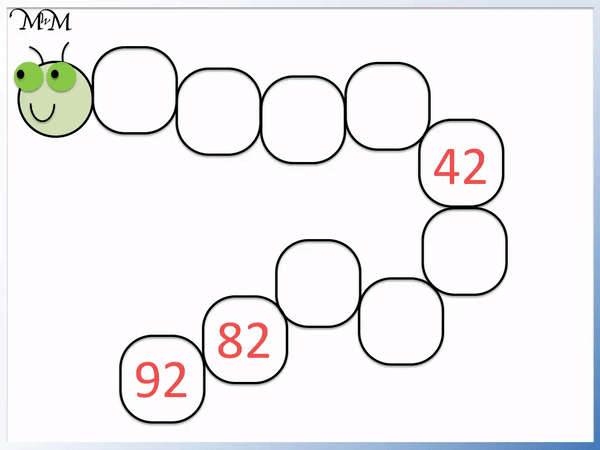92 ends in a ‘2’ in the units column. The coming numbers in the patter will also end in ‘2’ but the digit in the tens column will decrease by 1 each time.

The pattern learnt through this process allows us to then learn how to subtract ten from any number.

It helps us understand how the different numbers relate to each other in size.Now try our lesson on 2-Digit Column Addition where we learn how to add numbers using the column addition method.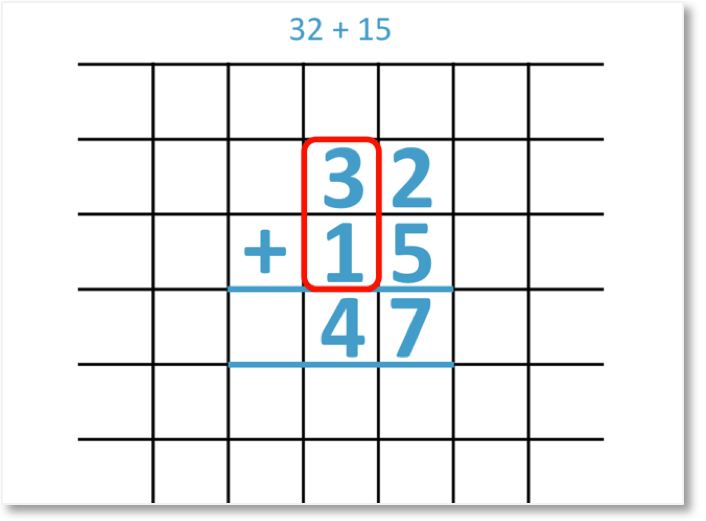error: Content is protected !!